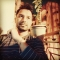## Find the smallest after deleting given elements using C++Published on 14-Feb-2022 09:21:12
In this problem, we are given two arrays arr[] and del[]. Our task is to find the smallest after deleting given elements.We will be deleting values from the array arr[] that are present in del[]. And then print the smallest value after deletion.Let’s take an example to understand the problem, ... Read More

## Find the slope of the given number using C++Published on 14-Feb-2022 09:16:48
In this problem, we are given a number N. Our task is to find the slope of the given number.Slope of a number is the total number of maxima and minima digits in the number.Maxima digit is the digit whose both neighbours (previous and next) are smaller.Maxima digit is the ... Read More

## Minimum Fibonacci terms with sum equal to K in C++Published on 14-Feb-2022 09:11:29
In this problem, we are given a number K. Our task is to find the Minimum Fibonacci terms with sum equal to K.Fibonacci Series generates subsequent numbers by adding two previous numbers. The Fibonacci series starts from two numbers − F0 & F1. The initial values of F0 & F1 ... Read More

## Find the number of elements greater than k in a sorted array using C++Published on 14-Feb-2022 08:04:13
In this problem, we are given an array arr[] consisting of N sorted integer values and an integer k. Our task is to Find the number of elements greater than k in a sorted array. Let’s take an example to understand the problem, Input arr[] = {1, 2, 5, 7, 8, ... Read More

## Maximum occurred integer in n ranges using C++Published on 14-Feb-2022 07:59:13
In this problem, we are given N ranges. Our task is to maximum occurred integer in n ranges.For the starting and ending value of all ranges. We need to find the value which occurs the most.Let’s take an example to understand the problem, Input S1 = 1, E1 = 3 ... Read More

## Find the Nth term of the series 14, 28, 20, 40,….. using C++Published on 14-Feb-2022 07:49:58
In this problem, we are given an integer value N.Our task is to find the nth term of the series −14, 28, 20, 40, 32, 64, 56, 112….Let’s take an example to understand the problem, InputN = 6Output64Solution ApproachTo find the Nth term of the series we need to find ... Read More

## C++ program to find Nth Non Fibonacci NumberPublished on 14-Feb-2022 07:43:34
In this problem, we are given an integer value N. Our task is to use a C + + program to find the Nth Non Fibonacci Number.Fibonacci Series generates subsequent number by adding two previous numbers. The Fibonacci series starts from two numbers − F0 & F1. The initial values ... Read More

## Find the row with maximum number of 1s using C++Published on 14-Feb-2022 07:30:35
In this problem, we are given a binary matrix where elements of each row are sorted. Our task is to find the row with maximum number of 1s.Let’s take an example to understand the problem, Inputmat[][] = {{ 0 1 1 1}    {1 1 1 1}    {0 0 ... Read More

## Find the repeating and the missing number using two equations in C++Published on 11-Feb-2022 13:06:01
In this problem, we are given an array arr[] of size N. It consists of integer values ranging from 1 to N. And one element x from the range is missing whereas one element y in the array occurs double. Our task is to find the repeating and the missing ... Read More

## Find the position of the last removed element from the array using C++Published on 11-Feb-2022 12:55:07
In this problem, we are given an array arr[] of size N and an integer value M. Our task is to find the position of the last removed element from the array.The removal of values from the array is based on the operations −For an element in the array arr[i]. ... Read More